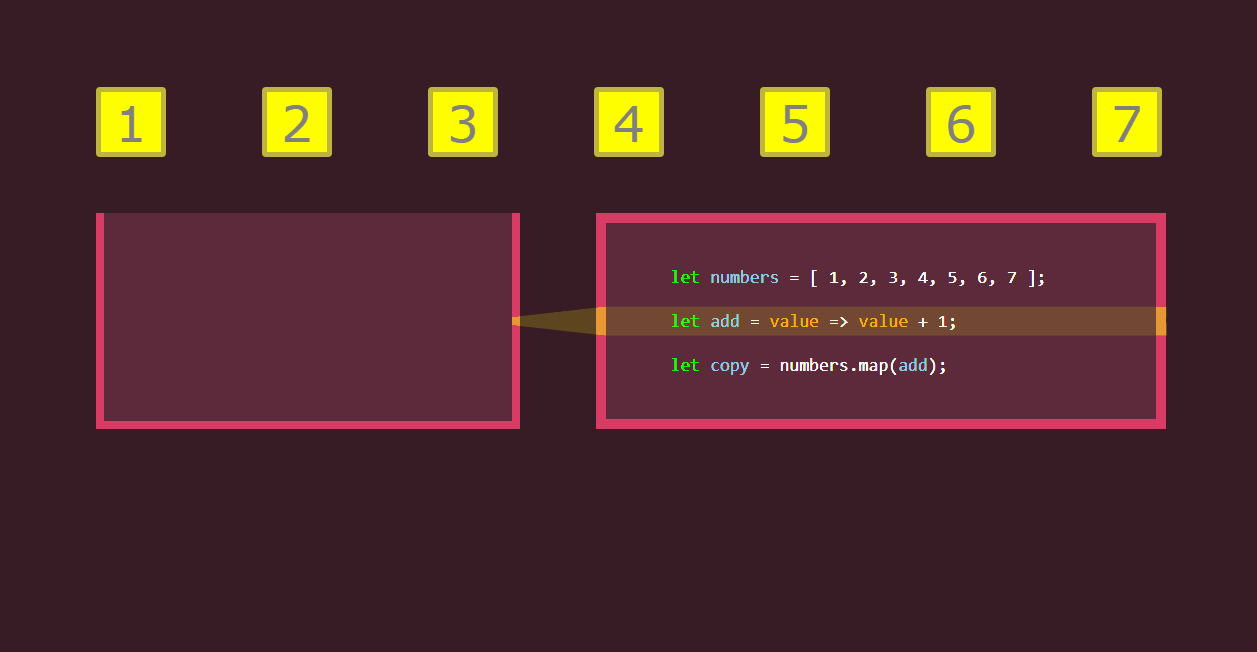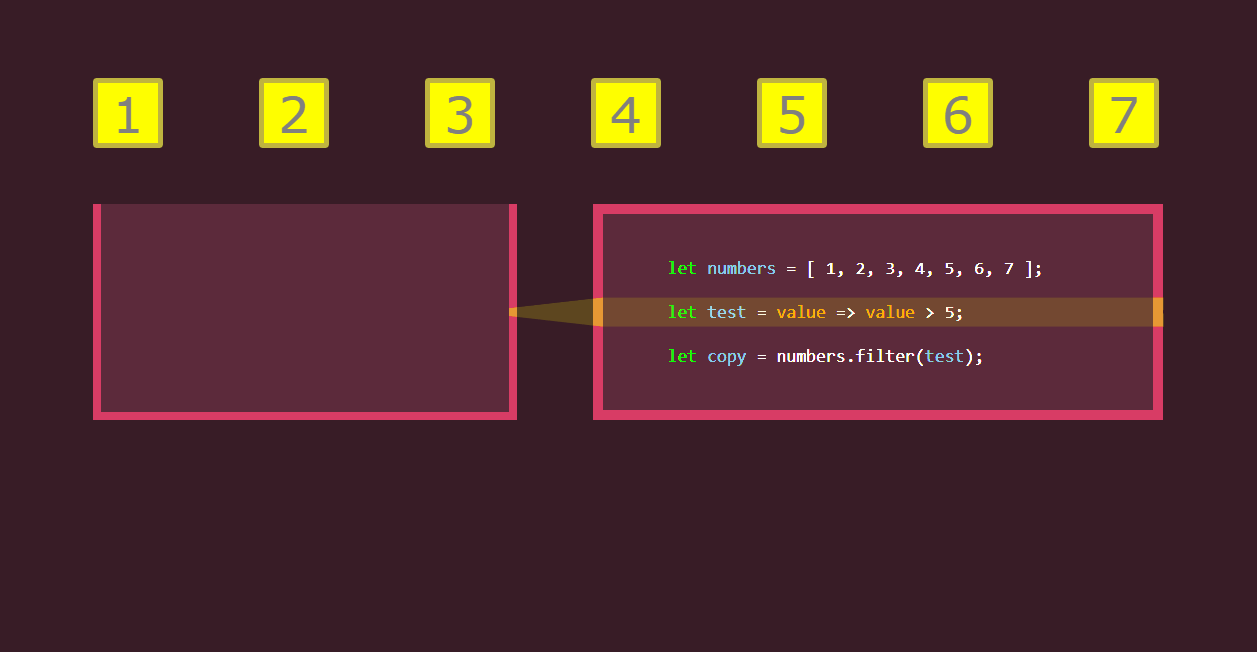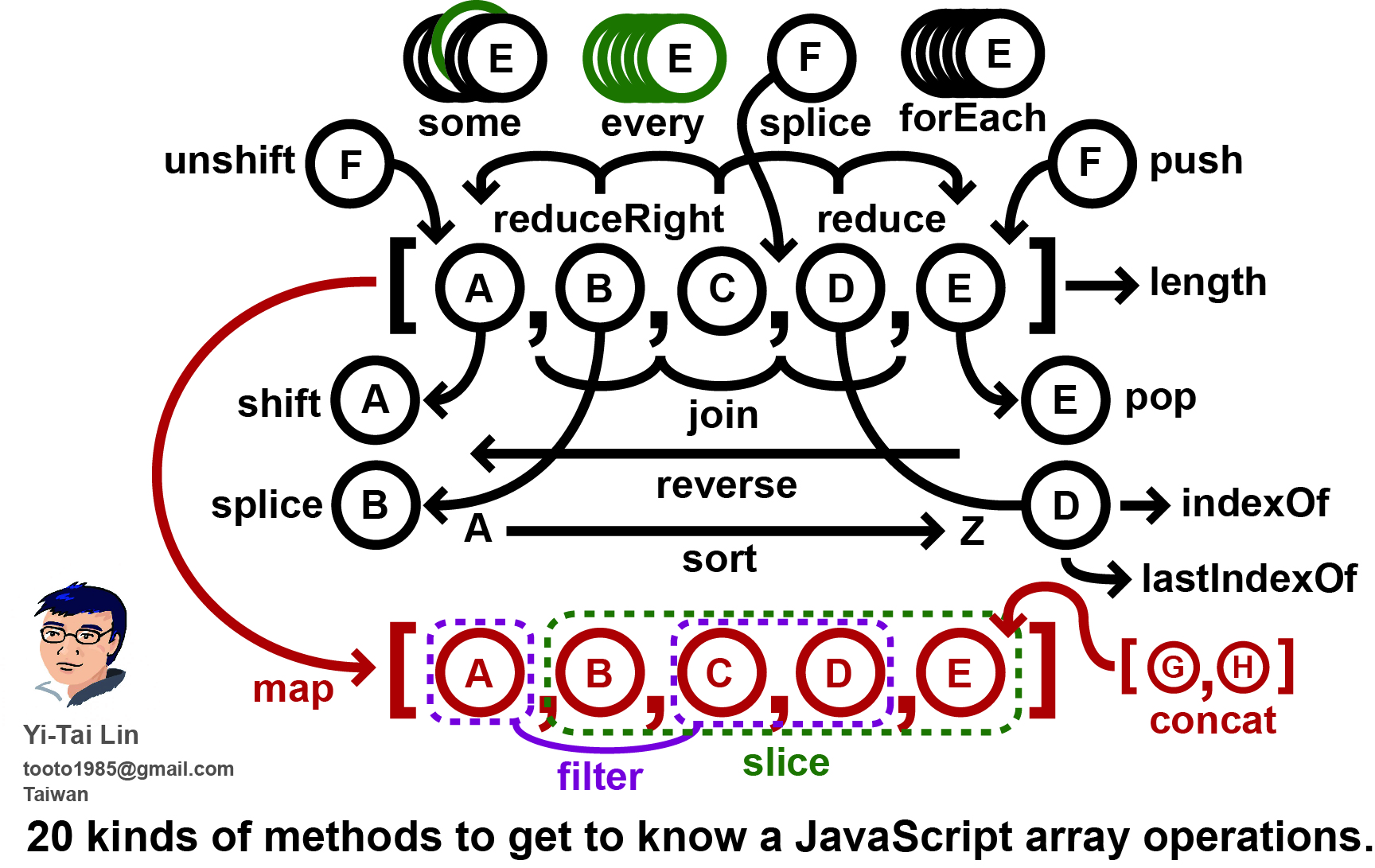# JavaScript基础7:数组Arrays常用的迭代方法

### 1、forEach()

`arr.forEach(callback[,thisArg]);`

callback：

• currentValue 数组中正在处理的当前元素。

• index  可选 数组中正在处理的当前元素的索引。

• array  可选  forEach() 方法正在操作的数组。

thisArg：

forEach 方法按升序为数组中含有效值的每一项执行一次callback 函数，那些已删除或者未初始化的项将被跳过。

```var arr=['成都','重庆','北京','上海'];
var city=[];
for(let i=0;i<arr.length;i++){
city.push(arr[i]);
}

//改成forEach()
arr.forEach(function(value){
city.push(value);
})```
```function logArray(value,index){
console.log('arr['+index+']='+value)
}
var arr=[1,2,,4,5,,7];
arr.forEach(logArray); //arr=1 arr=2 arr=4 arr=5 arr=7```

forEach 遍历的范围在第一次调用 callback 前就会确定。调用 forEach 后添加到数组中的项不会被 callback 访问到。如果已经存在的值被改变，则传递给 callback 的值是 forEach遍历到他们那一刻的值。已删除的项不会被遍历到。如果已访问的元素在迭代时被删除了（例如使用 shift()），之后的元素将被跳过。而且，不能中止遍历，除非报错。

```var arr=[1,2,-Infinity,'2',50];

arr.forEach(function(num,index){
if(num===-Infinity){
arr.splice(index,2);
}
console.log(num); //1,2,-Infinity
})

arr.forEach(function(num,index){
if(num===-Infinity){
arr.splice(index,2,Infinity,10);
}
console.log(num); //1,2,-Infinity,10,50
})```

```var oP=document.querySelectorAll('p');
console.log(oP); //NodeList(5) [p, p, p, p, p]
oP.forEach(function(obj,index){
obj.innerHTML=index;
});```

### 2、map()

```var arr=[2,4,8,10];
var newArr=arr.map(function(x){
return x/2;
})
console.log(newArr); //[1, 2, 4, 5]```

map里面的回调函数也可以不是自定义的函数，可以是现有的内置函数。

```var num=[2,15,'12','a'];
var newNum=num.map(isNaN); //isNaN是一个函数，所以不能加()。 [false, false, false, true]

var num=['1',2,'2.2e2',2.5e-3];
var newNum=num.map(Number);//[1, 2, 220, 0.0025]```

numString：必选项。要转换为数字的字符串。

radix :  可选项。在 2 和 36 之间的表示 numString 所保存数字的进制的值。如果没有提供，则前缀为 '0x' 的字符串被当作十六进制，前缀为 '0' 的字符串被当作八进制。所有其它字符串都被当作是十进制的。

parseInt 方法返回与保存在 numString 中的数字值相等的整数。如果 numString 的前缀不能解释为整数，则返回 NaN（而不是数字）。

```var num=[1,2,10,4,5,6,12,16,7];
var newNum=num.map(parseInt); // [1, NaN, 2, NaN, NaN, NaN, 8, 13, 7]```
```parseInt(10,2);// 2
parseInt('0xffffff',16); //16777215
parseInt(7,8); //7
parseInt(2,10);//2```

`obj1.(method).call(obj2,argument1,argument2)`

call()方法就是把obj1的方法拿给obj2用。argument是obj1方法的参数。

```<label><input type="checkbox" name="hobby" id="c1" value="编程">编程</label>
<label></label><input type="checkbox" name="hobby" id="c2" checked value="美术">美术</label>
<label></label><input type="checkbox" name="hobby" id="c3" checked value="阅读">阅读</label>
<script>
var oCheckbox=document.querySelectorAll("input:checked"); //NodeList(2) [input#c2, input#c3]
var values=Array.prototype.map.call(oCheckbox,function(obj){
return obj.value;
})
console.log(values); //["美术", "阅读"]
</script>```### 3、filter()

filter是过滤，筛选的意思，顾名思义，应该是数组元素挨个进行某个条件测试，然后返回成功通过的新数组

`var newArray = arr.filter(callback(element[, index[, array]])[,thisArg])`

callback```var age=[12,18,25,45,60,17,22];
var result=age.filter(function(value){
return value>=18 && value<=45;
})
console.log(result); //[18, 25, 45, 22]```
```var arr=[2,8,'a','12',-12,null,undefined,,'',NaN];

function myFilter(value){
return  value!==undefined&&typeof(value)==='number'&&!isNaN(value)&&value!==0;
}
var newArr=arr.filter(myFilter);
console.log(newArr); // [2, 8, -12]```

### 4、every()

```function isBig(value) {
return value >= 60 && value <= 100;
}
var result = [85, 75, 98, 53, 60, 105].every(isBig);
console.log(result); //false

[85, 75, 98, 53, 60, 105].every(value=>value>=60&&value<=100)```

### 5、some()

• 如果一个元素满足条件,返回true,且后面的元素不再被检测

• 所有元素都不满足条件，则返回false

• 不会改变原始数组

• 不会对空数组进行检测;数组为空的话，直接返回false

```function isBiggerThan10(item){
return item>10;
}
[12,10,8,7,-5].some(isBiggerThan10); //true;```

```var myname=['老赵','小何','小王'];
function isExit(arr,val){
return  arr.some(function(value){
return value===val;
})
}
console.log(isExit(myname,'老赵')); //true```

### 6、includes()

ES6的新方法，Edge14及以上才支持。

`arr.includes(valueToFind[,fromIndex])`

```[1, 2, 3].includes(2);     // true
[1, 2, 3].includes(4);     // false
[1, 2, 3].includes(3, 3);  // false
[1, 2, 3].includes(3, -1); // true
[1, 2, 3].includes(3, -4); // true
[1, 2, NaN].includes(NaN); // true```

```// array length is 3
// fromIndex is -100//
computed index is 3 + (-100) = -97
var arr = ['a', 'b', 'c'];
arr.includes('a', -100); // true
arr.includes('b', -100); // true
arr.includes('c', -100); // true
arr.includes('a', -2); // false```

`[NaN].indexOf(NaN);// -1`

```[NaN].includes(NaN);// true
[-0].includes(0);// true```

```const contains = (() =>
Array.prototype.includes
? (arr, value) => arr.includes(value)
: (arr, value) => arr.some(el => el === value)
)();
contains(['foo', 'bar'], 'baz'); // => false```

### 7、find()

`arr.find(callback(element[, index[, array]])[, thisArg])`

Edge12及以上支持，IE9不支持。

```const myArr=[1,2,3,4,5,6];
var v=myArr.find(value=>value>4);
console.log(v);// 5

const myArr=[1,2,3,4,5,6];
var v=myArr.find(value=>value>40);
console.log(v);// undefined```

### 8、findIndex()

`arr.findIndex(callback(element[, index[, array]])[, thisArg])`

```const students=[
{
id:1,
s_name:'小王'
},
{
id:2,
s_name:'小赵'
},
{
id:3,
s_name:'小李子'
}
]
var i=students.findIndex(value=>value.id==2);
console.log(i); //1
var n=students.findIndex(value=>value.id==5);
console.log(n); //-1```

```var arr1=[NaN,undefined,null,2,0];
console.log(arr1.indexOf(NaN)); //-1
console.log(arr1.includes(NaN)); //true
console.log(arr1.find(function(value){ //null
return value===null;
}));
console.log(arr1.findIndex(function(value){ //1
return value===undefined;
}))
console.log(arr1.filter(function(value){ // [null, 2, 0]
return value>=0;
}))```

### 9、reduce()

reduce() 方法对数组中的每个元素执行一个回调函数中提供的reducer函数(升序执行)，将其结果汇总为单个返回值。

`arr.reduce(callback(accumulator, currentValue[, index[, array]])[, initialValue])`

reducer 函数接收4个参数:

• Accumulator (acc) (累计器) 累计器累计回调的返回值; 它是上一次调用回调时返回的累积值，或initialValue（初始值）。

• Current Value (cur) (当前值) 数组中正在处理的元素。

• Current Index (idx) (当前索引)  数组中正在处理的当前元素的索引。 如果提供了initialValue，则起始索引号为0，否则为1。

• Source Array (src) (源数组)  调用reduce()的数组

reducer 函数的返回值分配给累计器，该返回值在数组的每个迭代中被记住，并最后成为最终的单个结果值。

initialValue 可选 作为第一次调用 callback函数时的第一个参数的值。 如果没有提供初始值，则将使用数组中的第一个元素。 在没有初始值的空数组上调用 reduce 将报错。

```var sum = [0, 1, 2, 3].reduce(function (accumulator, currentValue) {
return accumulator + currentValue;}, 0);
//6```
```var initialValue = 0;var sum = [{x: 1}, {x:2}, {x:3}].reduce(function (accumulator, currentValue) {
return accumulator + currentValue.x;},initialValue)
console.log(sum)
// logs 6```
```var flattened = [[0, 1], [2, 3], [4, 5]].reduce(
function(a, b) {
return a.concat(b);
},
[]);
// flattened is [0, 1, 2, 3, 4, 5]```1

### 相关文章

◎请发表你卖萌撒娇或一针见血的评论，严禁小广告。

Top